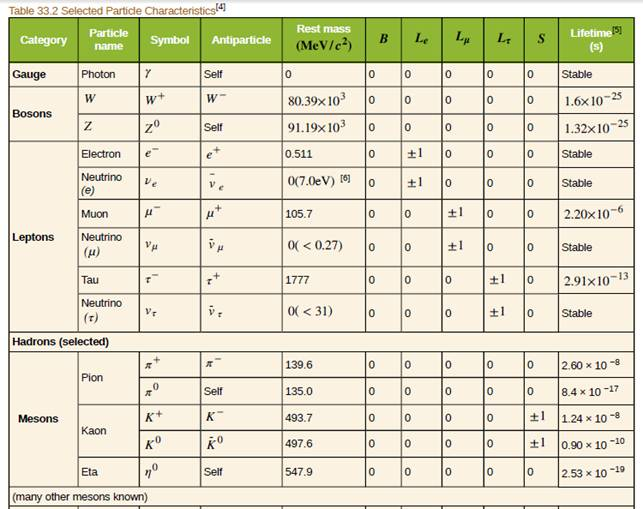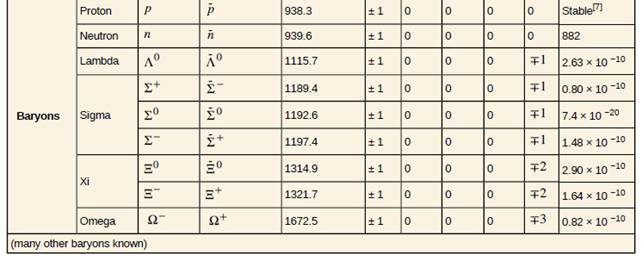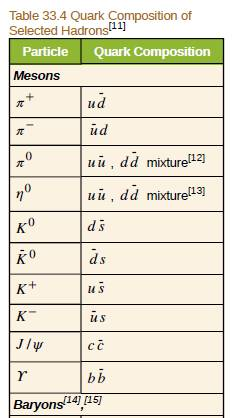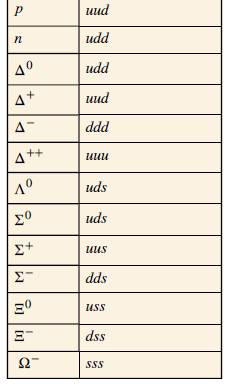Chapter 33, Problem 36PE

Chapter
Section
Textbook Problem

Verify the quantum numbers given for the proton and neutron in Table 33.2 by adding the quantum numbers for their quark constituents as given in Table 33.4.To determine

To verify the quantum numbers of proton and neutron with the help of quark structure.

Explanation

Given info:

Quarks are fundamental particles and the quark structure of proton is uud and that of neutron is udd

Calculation:

For proton, B=+1 , S=0 and charge =+e

We can verify this quantum numbers with the help of quarks.

Quark structure of proton is uud.

Baryon number of quark u is 13 and that of quark d is also 13.

So, baryon number of proton, =13+13+13=+1

Charge of quark u=+23e and that of quark d=13e

So, charge of proton =23e+23e+13e=+e

Strangeness number of quark, u=0 and that of quark d=0

So, strangeness number of proton, =0+0+0=0

Similarly, for neutron, B=+1 , S=0 and charge =0

We can verify this quantum numbers with the help of quarks

Still sussing out bartleby?

Check out a sample textbook solution.

See a sample solution

The Solution to Your Study Problems

Bartleby provides explanations to thousands of textbook problems written by our experts, many with advanced degrees!

Get Started